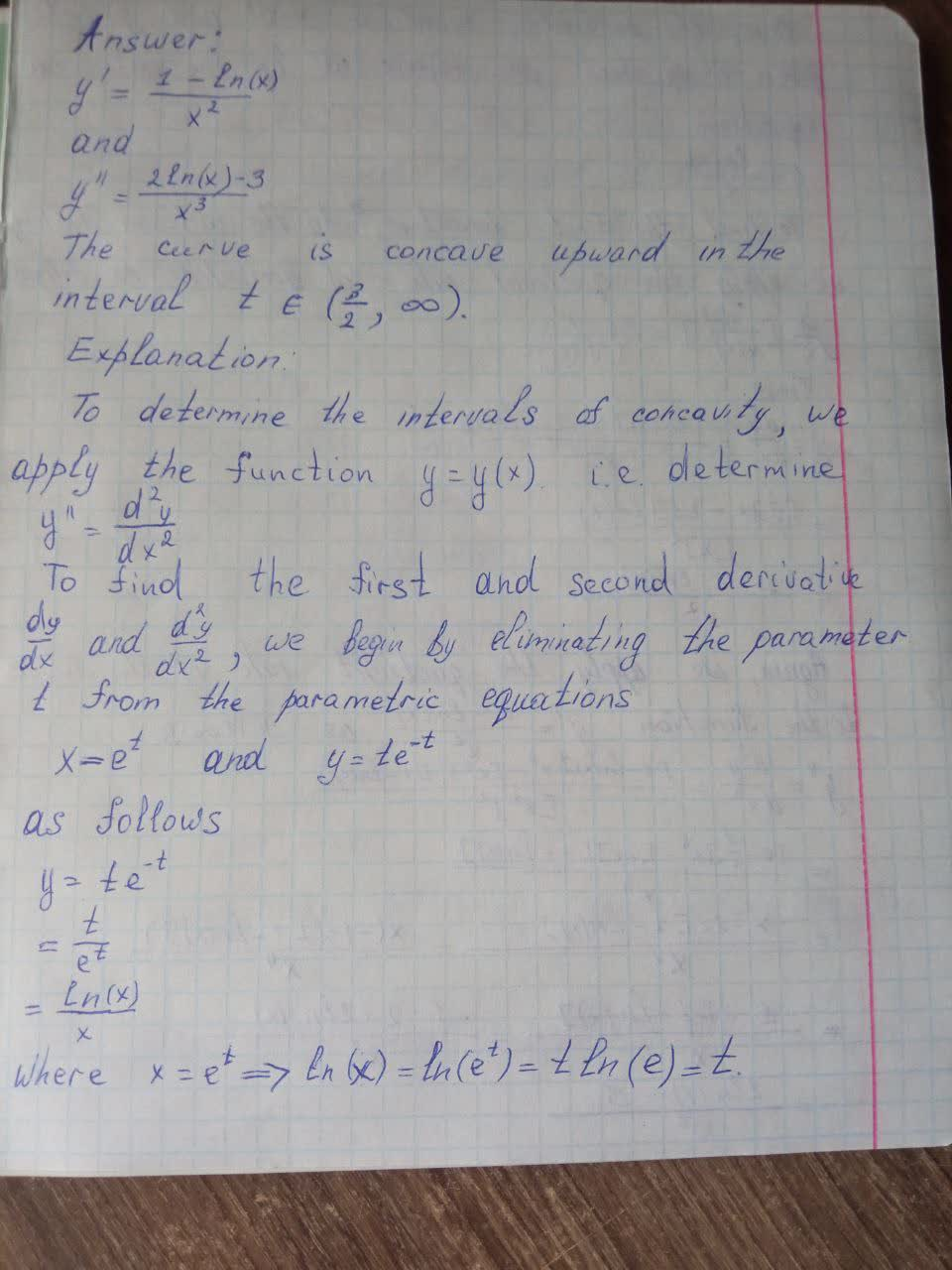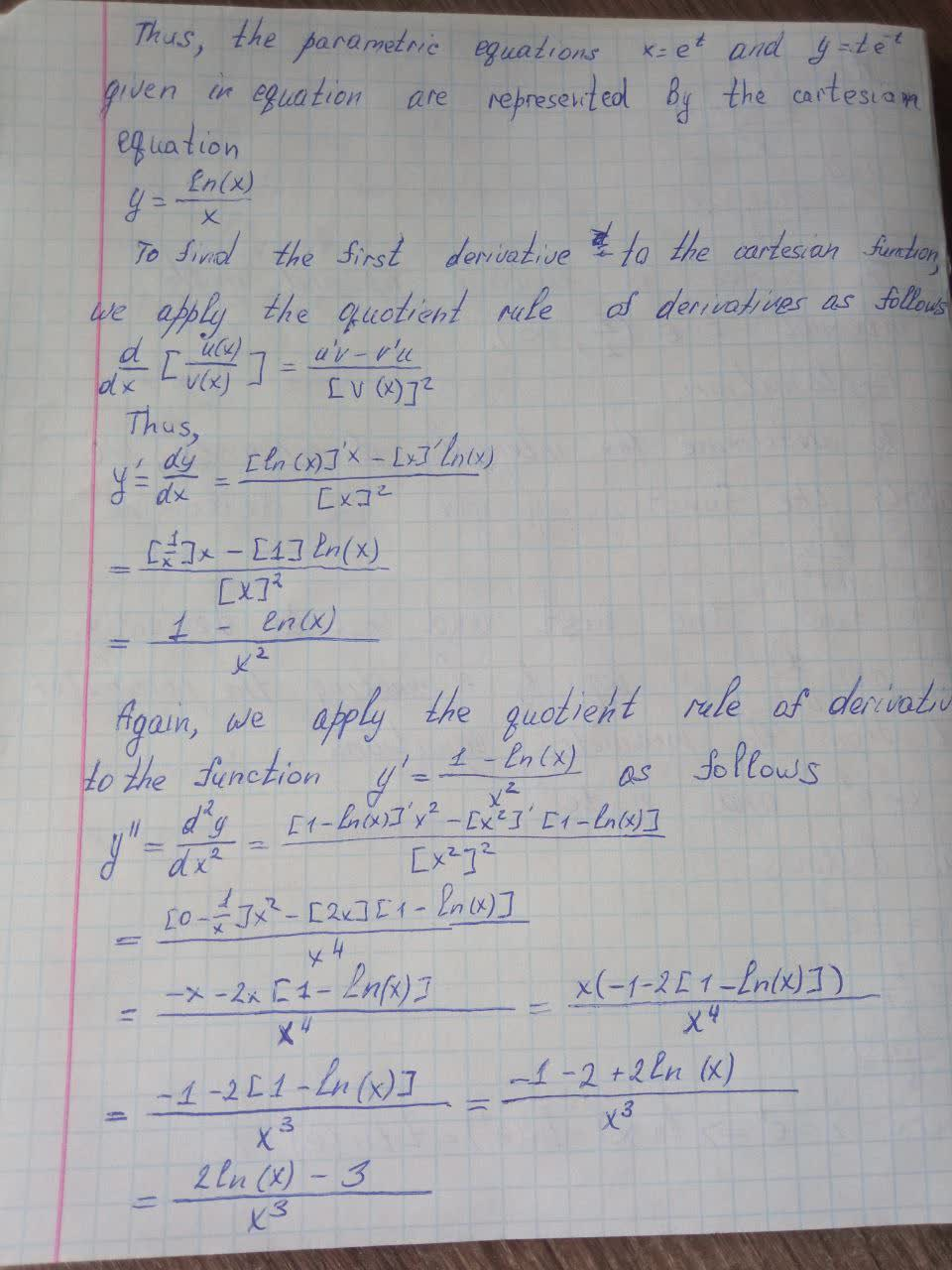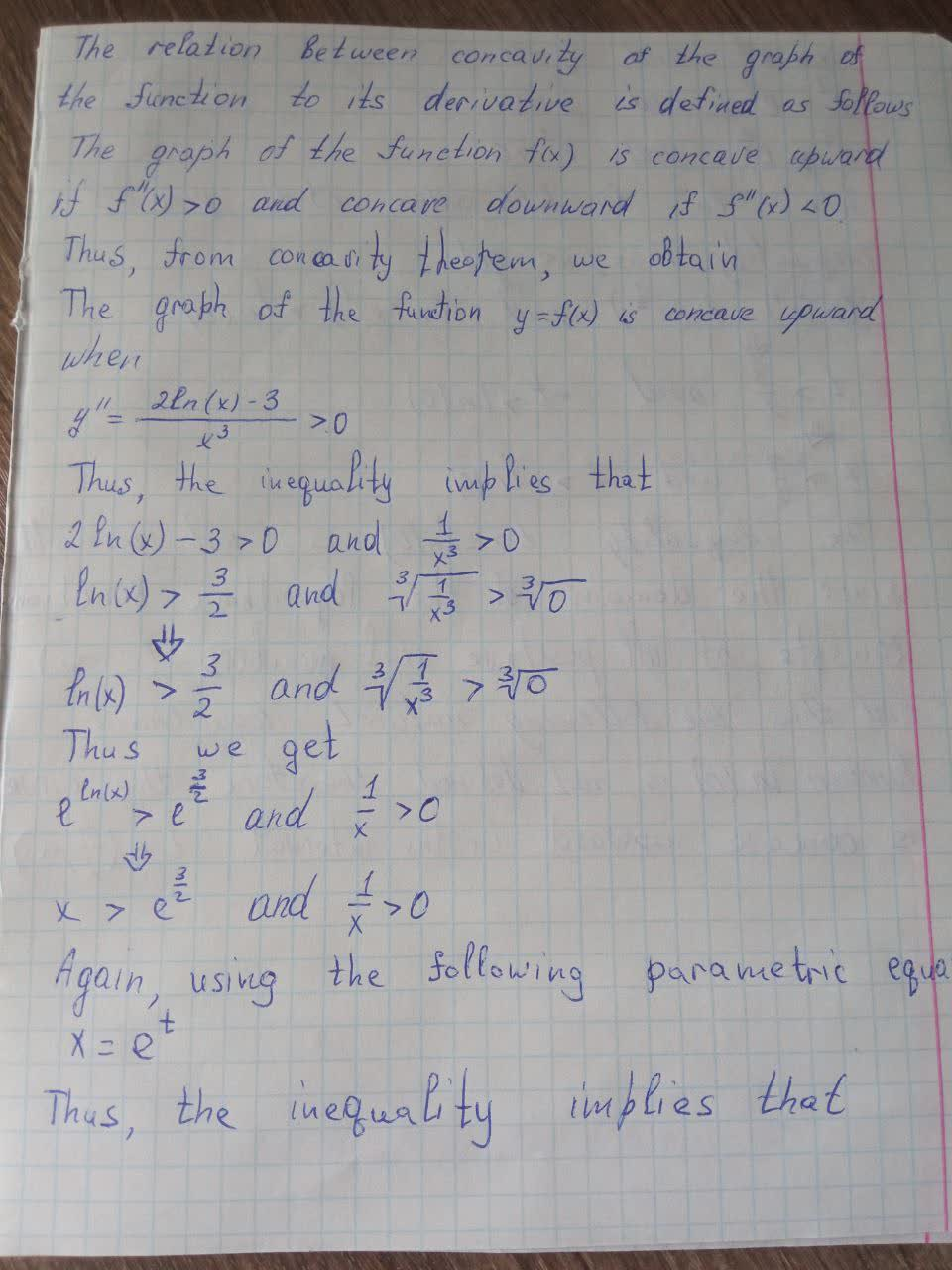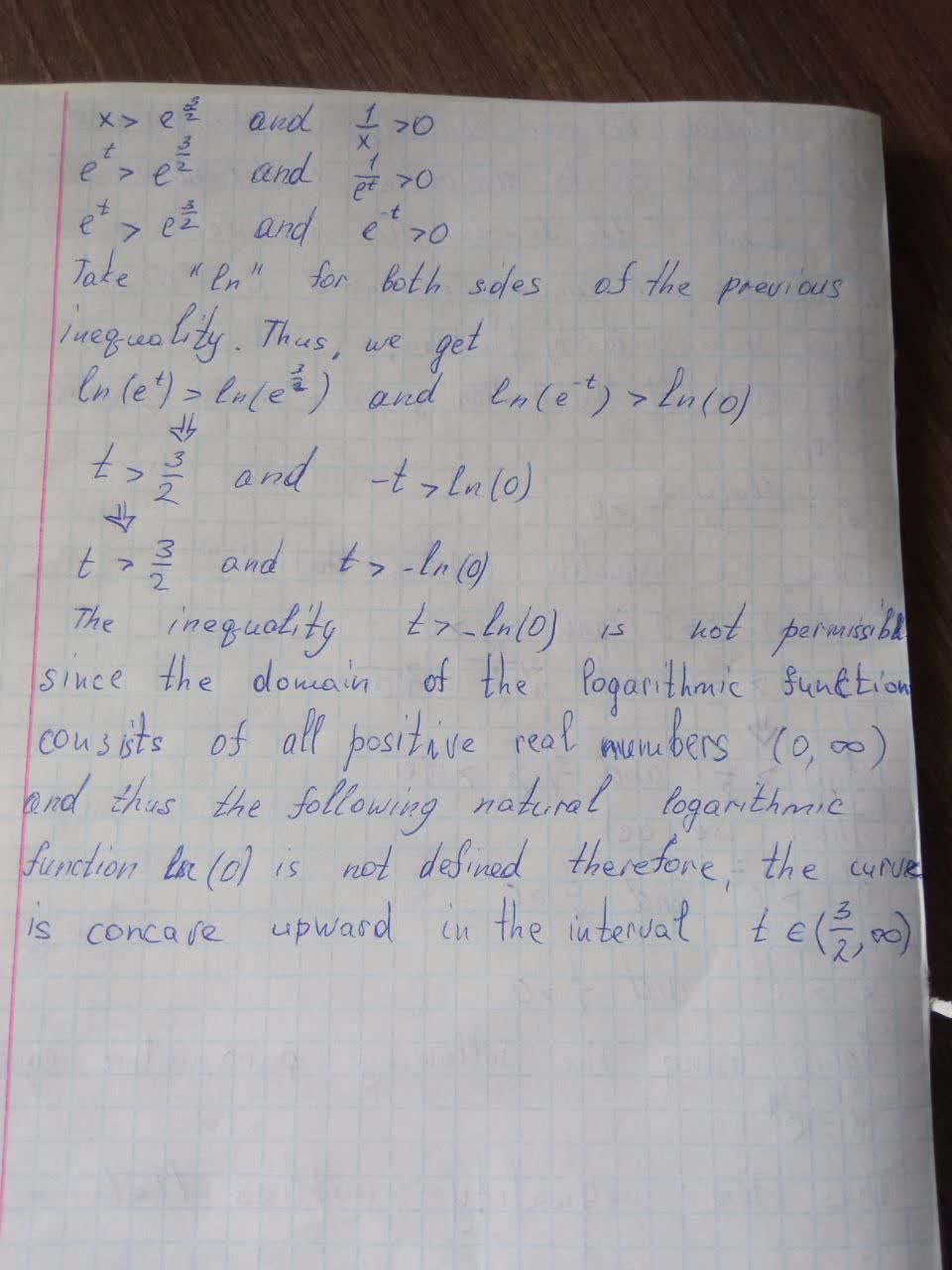Question# Find \frac{dy}{dx} and \frac{d^2y}{dx^2}.x=e^t,y=te^{-t}. For which values of t is the curve concave upward?

Differential equations
ANSWEREDFind $$\frac{dy}{dx}$$ and $$\frac{d^2y}{dx^2}.x=e^t,y=te^{-t}$$. For which values of t is the curve concave upward?2021-05-042021-09-29

$$y'=\frac{1-\ln x}{x^2}$$

and

$$y''=\frac{2\ln(x)-3}{x^3}$$

The curve is concave upward in the interval $$t\in(\frac{3}{2},\infty)$$

Explanation:

To determine the intervals of concavity, we apply the second derivative test to the function $$y=y(x)$$, i.e. determine $$y''=\frac{d^2y}{dx^2}$$

To find the first and second derivative $$\frac{dy}{dx}$$ and $$\frac{d^2y}{dx^2}$$

as follows

$$y=te^{-t}$$

$$=\frac{t}{e^t}$$

$$=\frac{\ln(x)}{x}$$

where $$x=e^t\Rightarrow\ln(x)=\ln(e^t)=t\ln(e)=t$$

Thus, the parametric equations $$x=e^t$$ and $$y=te^{-t}$$ given equation (1) are represented by the cartesian equation (1) are represented by the cartesian equation

$$y=\frac{\ln x}{x}$$

where $$x=e^t\Rightarrow\ln(x)=\ln(e^t)=t\ln(e)=t$$

Thus, the parametric equations $$x=e^t$$ and $$y=te^{-t}$$ given in equation are represented by the cartesian equation

$$y=\frac{\ln(x)}{x}$$

To find the first derivative to the cartesian function we apply the quotient rule of derivatives as follows

$$\frac{d}{dx}[\frac{u(x)}{v(x)}]=\frac{u'v-v'u}{[v(x)]^2}$$

Thus,

$$y'=\frac{dy}{dx}=\frac{[\ln(x)]'x-[x]'\ln(x)}{[x]^2}$$

$$=\frac{[\frac{1}{x}]x-\ln(x)}{[x]^2}$$

$$=\frac{1-\ln(x)}{x^2}$$

Again, we apply the quotient rule of derivatives to the function $$y'=\frac{1-\ln(x)}{x^2}$$ as follows

$$y''=\frac{d^2y}{dx^2}=\frac{[1-\ln(x)]'x^2-[x^2]'[1-\ln(x)]}{[x^2]^2}$$

$$=\frac{[0-\frac{1}{x}]x^2-[2x][1-\ln(x)]}{x^4}$$

$$=\frac{-x-2x[1-\ln(x)]}{x^4}=\frac{x(-1-2[1-\ln(x)])}{x^4}$$

$$=\frac{-1-2[1-\ln(x)]}{x^3}=\frac{-1-2+2\ln(x)}{x^3}$$

$$=\frac{2\ln(x)-3}{x^3}$$

The relation the concavity of the graph of the function to its derivative is defined as follows

The graph of the function $$f(x)$$ is concave upward if $$f''(x)>0$$ and concave downward if $$f''(x)<0$$

Thus, from concavity theorem, we obtain

The graph of the function $$y=f(x)$$ is concave upward when

$$y''=\frac{2\ln(x)-3}{x^3}>0$$

Thus, the inequality implies that

$$2\ln(x)-3>0$$ and $$\frac{1}{x^3}>0$$

$$\ln(x)>\frac{3}{2}$$ and $$\sqrt{\frac{1}{x^3}}>\sqrt{0}$$

$$\ln(x)>\frac{3}{2}$$ and $$\sqrt{\frac{1}{x^3}}>\sqrt{0}$$

Thus we get

$$e^{\ln(x)}>e^{\frac{3}{2}}$$ and $$\frac{1}{x}>0$$

$$x>e^{\frac{3}{2}}$$ and $$\frac{1}{x}>0$$

Again, using the following parametric equation

$$x=e^t$$

Thus, the inequality implies that

$$x>e^{\frac{3}{2}}$$ and $$\frac{1}{x}>0$$

$$e^t>e^{\frac{3}{2}}$$ and $$\frac{1}{e^t}>0$$

$$e^t>e^{\frac{3}{2}}$$ and $$e^{-t}>0$$

Take "ln" for both sides of the previous inequality. Thus we get

$$\ln(e^t)>\ln(e^{\frac{3}{2}})$$ and $$\ln(e^{-t})>\ln(0)$$

$$t>\frac{3}{2}$$ and $$-t>\ln(0)$$

$$t>\frac{3}{2}$$ and $$t>-\ln(0)$$

The inequality $$t>-\ln(0)$$ is not permissible since the domain of the logarithmic function consists of all positive real numbers $$(0,\infty)$$ and thus the following natural logarithmic function $$\ln(0)$$ is not defined.

therefore, the curve is concave upward in the interval $$t\in(\frac{3}{2},\infty)$$